Formula Column

Zoho Analytics allows you to define your own powerful formulas to meet your specific reporting requirements. This enables you to easily perform from simple calculations such as addition and subtraction to a complex combination of in-built functions. As the output of the formula adds a new column in the table is called a Formula Column. You can use these formula columns in creating reports in the same way you use other columns in a table.

Creating Formula Column

Zoho Analytics provides you with a variety of in-built functions which are predefined mathematical formulas which are designed to perform specific well-known calculations easily. These in-built functions can be used in combination with the table columns and basic arithmetic operators like +, -, / and * to create your formula column.

To create and use formula columns:

• From the Explorer tab of the Workspace, select the table to which you want to add the formula column.
• Select Add > Formula Column option from the toolbar or right click on a column in the table and select  Add Formula > Formula Column.
• The Formula Column  dialog box will open as shown below. This dialog box provides easy access to the names of the columns in your table, as well as to many of the in-built functions that are available to create formulas. A brief description of the function with syntax and an example will be displayed at the bottom of the dialog box when you hover your mouse over a function.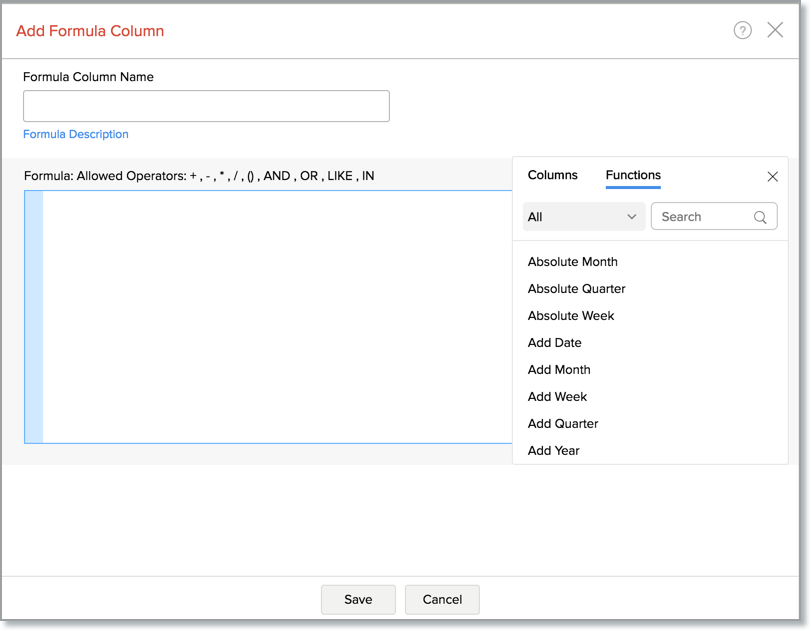• In the Add Formula Column dialog box, select the columns and functions that you want to insert from the list under the Click to Insert Columns and the Click to Insert Function boxes.

For example, to build a formula column that adds particular number of days to a given date:

• Select Add Date function under the Click to Insert Functions box. You will notice that adddate() syntax appears in the Formula text box.
• In the Formula text box, position the cursor in between the parenthesis, select the date column to which you wanted to add date using the Click to Insert Columns option.
• Enter the number of days (say 10) that you want to add separated by a comma.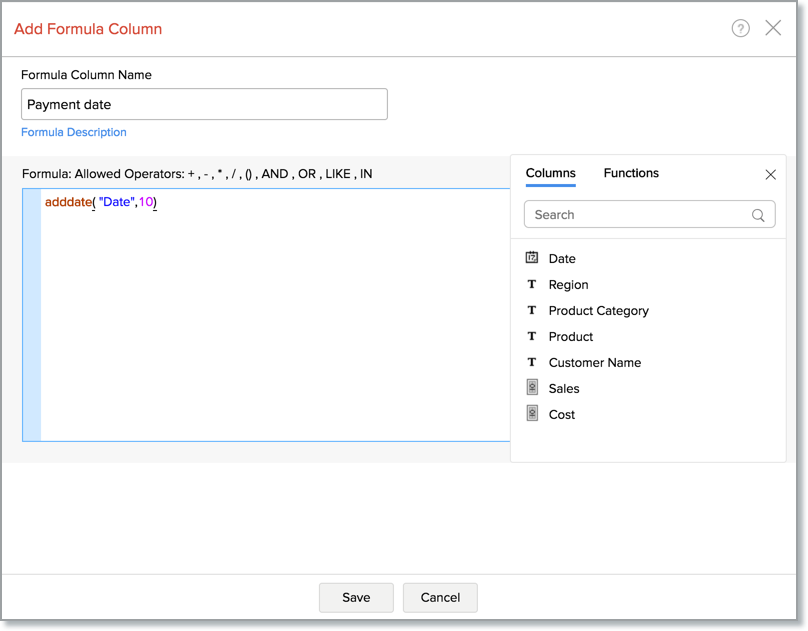To create a formula column with this expression, type column name in the Formula Column Name text box and then click OK. A new formula column will be created in your table as shown below.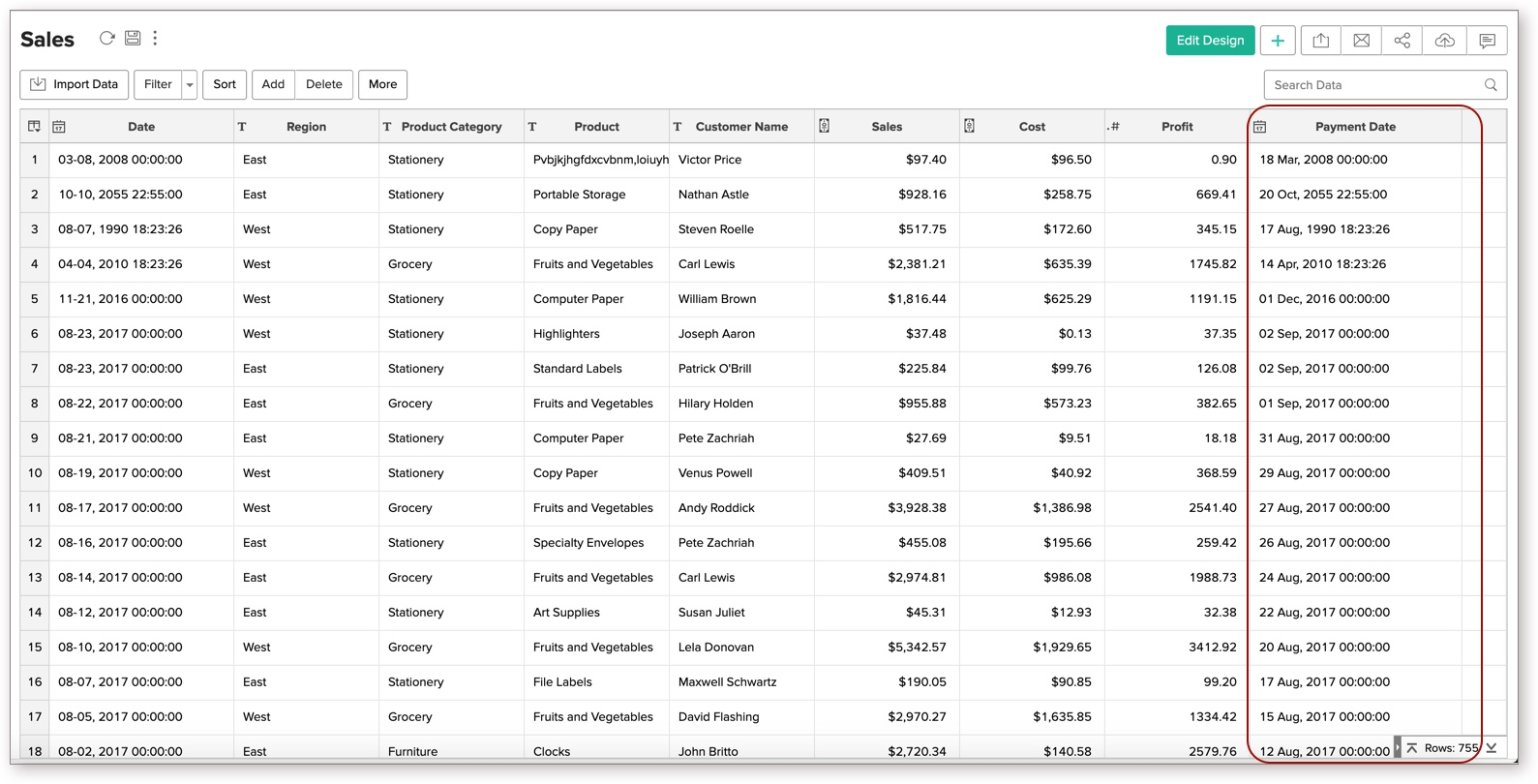You can edit the formula column any time by right clicking on the formula column listed in the table and clicking Edit Formula Column in the popup menu. Also refer to View/Edit Formulas to know how you could view all the formulas defined on a table and handle edition or deletion of the same.

Quick Add option for Formula Columns

Zoho Analytics provides an easy and convenient way to add formula columns which are based on a limited set of widely used functions. You can find this quick add option as follow.

• Select a column based on which you want to add the formula column.
• Zoho Analytics automatically identifies the data type of the column selected and displays a list of widely used pre-defined formulas which are applicable to that as shown in the screenshot below.
• On selecting the required formula from the list, Zoho Analytics applies the formula on the corresponding column and adds a new formula column, at the right end of the table.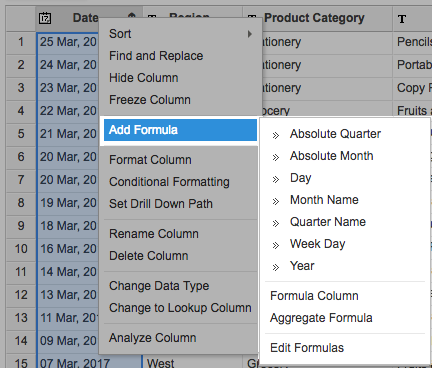In-built functions provided by Zoho Analytics

Zoho Analytics provides you with a variety of in-built functions which are predefined mathematical formulas designed to perform specific well-known calculations easily. Refer to the In-Built Function page to view the complete list of functions.

Creating Formulas Over Formula Column

Formula columns are similar to other columns of the table. You can make use of existing formula columns while creating a new formula column, as you do with other columns of the table. Reusing existing formulas column to create new formula columns helps you create powerful formula combinations, as well as eases in the maintenance of this formula structure in your Workspace. Refer to the above section to know how to create formula column.

Creating Reports Using Formula Column

Formula columns can be used while creating reports as you would use a column in a table. When you create a new report over the table, in which you have added the formula column, all these formula columns will be listed in the Columns list pane on the left, which can be drag and drop to create the report. You can apply functions on the formula column, as you do for other columns, using the combo box that is displayed over the column when dropped in the design area of the report.

The following screenshot shows the Payment Date formula column listed in the columns list page: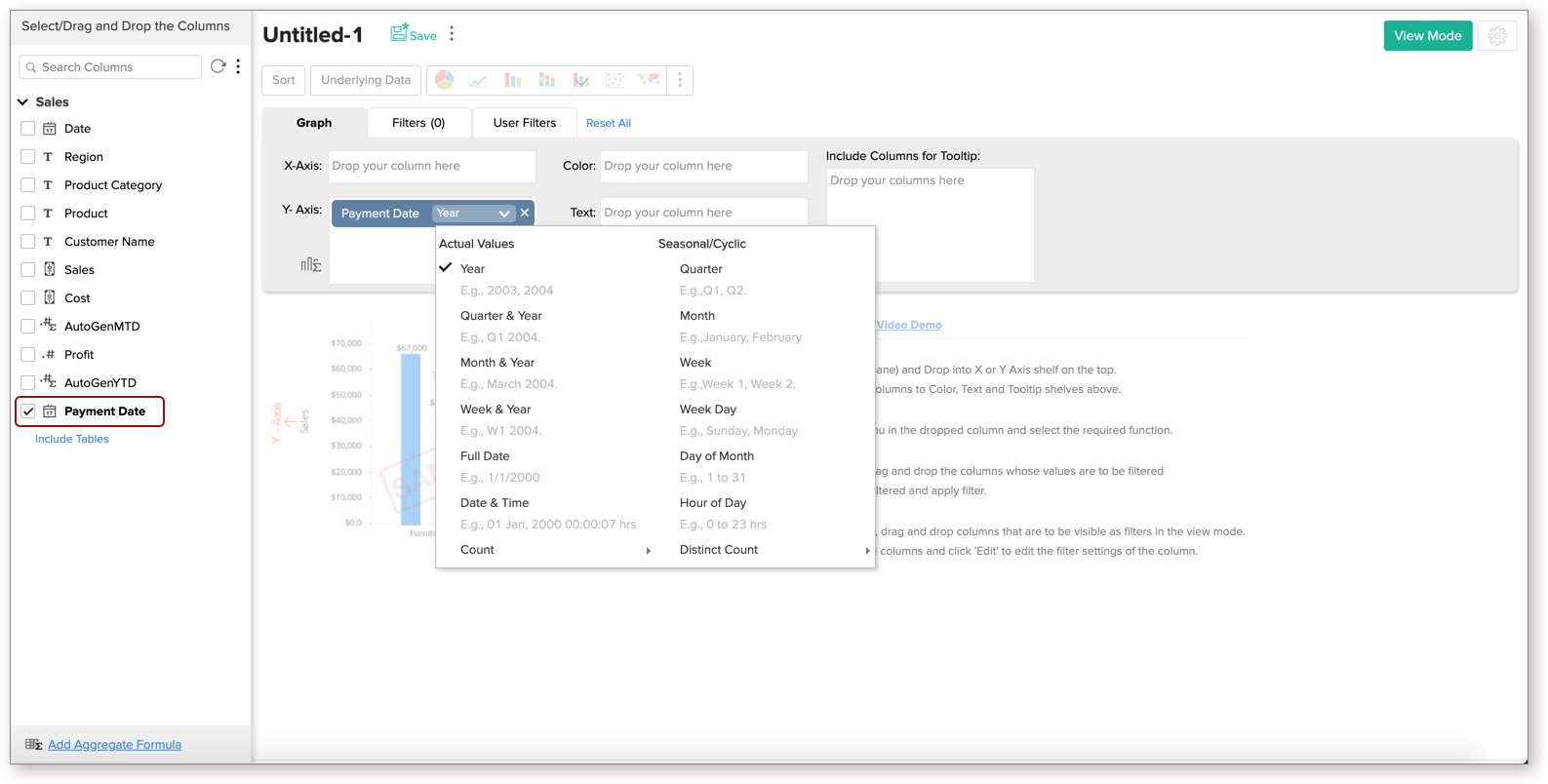Sharing a Formula Column

Once a Formula column is created, it behaves like any other column in a table. When you share a table, all the existing formula columns will also be shared to the user.

Note:

• The shared user will not be able to edit, delete or format the shared formulas. Also the shared user will not be able to add his own formula column over the shared table, unless the shared user is a Workspace Administrator.

Sharing Aggregate Formulas

With respect to sharing and collaboration, Aggregate Formulas behave similarly to how a column in a table behaves. When you share a table, all the aggregate formulas that are created over the table will be also shared to the user to whom you are sharing the table. Hence the shared user will be able to use these formulas while creating any report over the data table.

Note:

• The shared user will not be able to edit, delete or format the shared aggregate formula, but can just use it in report creation. Also, the shared user will not be able to add his own Aggregate formulas over the shared table, unless the user is a  Workspace Administrator.

View/Edit Formulas

Zoho Analytics allows you to view, edit, format and delete all the formulas defined for a table through the Edit Formula option. You can view & edit both Formula Columns and Aggregate Formulas using this option. To view/edit existing Formula Column or Aggregate Formulas:

• Select the required table.
• Select Add > Edit Formulas option from the toolbar.
• The Edit Formula dialog box with a list of all the formulas associated to the table will open as shown below: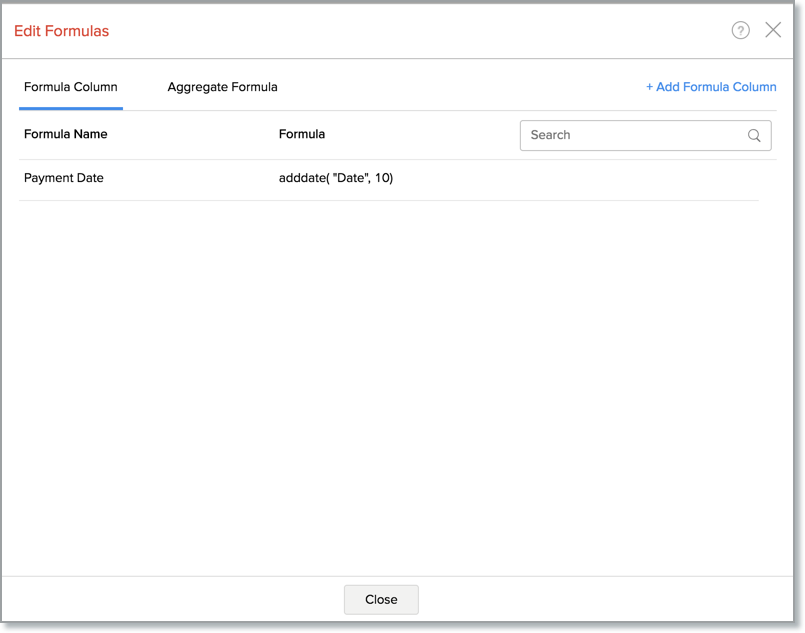• Click Edit link next to the formula that you wish to edit.
• The Edit Formula dialog box will open, you can modify the existing formula the same way as you would create a new formula.
• Edit Formula: You can edit the constructed formula using the Edit link provided next to the corresponding formula.
• Delete Formula: You can delete an existing Formula any time from the table using the Remove link provided next to the corresponding formula.
• Format Formula: You can change the format of the Formula result, like alignment, decimal places, date format, currency symbols etc., depending on its data type of the formula using the Format link provided. It's similar to how you format a data column. Refer here to know how to format a column.